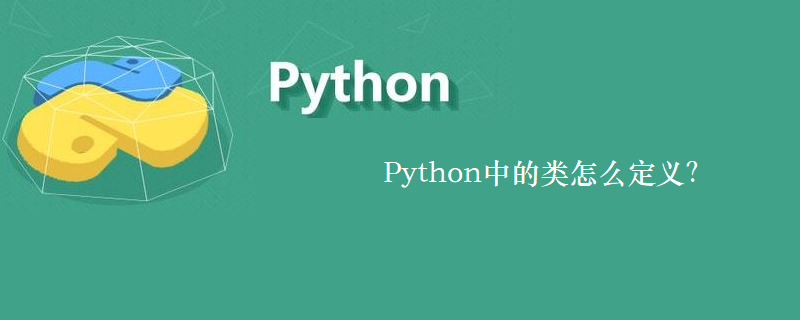## Python中的类怎么定义？

262次阅读

Python中的类定义：

```class <类名>:
<语句>```__private_attrs 两个下划线开头，声明该属性为私有，不能在类地外部被使用或直接访问。在类内部的方法中使用时 self.__private_attrs

在类地内部，使用def关键字可以为类定义一个方法，与一般函数定义不同，类方法必须包含参数self,且为第一个参数

__private_method 两个下划线开头，声明该方法为私有方法，不能在类地外部调用。在类的内部调用slef.__private_methods

__init__ 构造函数，在生成对象时调用

__del__ 析构函数，释放对象时使用

__repr__ 打印，转换

__setitem__按照索引赋值

__getitem__按照索引获取值

__len__获得长度

__cmp__比较运算

__call__函数调用

__sub__减运算

__mul__乘运算

__div__除运算

__mod__求余运算

__pow__称方

```#类定义
class people:
#定义基本属性
name = ''
age = 0
#定义私有属性,私有属性在类外部无法直接进行访问
__weight = 0
#定义构造方法
def __init__(self,n,a,w):
self.name = n
self.age = a
self.__weight = w
def speak(self):
print("%s is speaking: I am %d years old" %(self.name,self.age))
p = people('tom',10,30)
p.speak()```

1.单继承

```class <类名>(父类名)
<语句>```

```class childbook(book)
age = 10```

```#单继承示例
class student(people):
def __init__(self,n,a,w,g):
#调用父类的构函
people.__init__(self,n,a,w)
#覆写父类的方法
def speak(self):
print("%s is speaking: I am %d years old,and I am in grade %d"%(self.name,self.age,self.grade))
s = student('ken',20,60,3)
s.speak()```

2.类的多重继承

```class 类名(父类1,父类2,....,父类n)
<语句1>```

```#另一个类，多重继承之前的准备
class speaker():
topic = ''
name = ''
def __init__(self,n,t):
self.name = n
self.topic = t
def speak(self):
print("I am %s,I am a speaker!My topic is %s"%(self.name,self.topic))
#多重继承
class sample(speaker,student):
a =''
def __init__(self,n,a,w,g,t):
student.__init__(self,n,a,w,g)
speaker.__init__(self,n,t)
test = sample("Tim",25,80,4,"Python")
test.speak()#方法名同，默认调用的是在括号中排前地父类的方法```